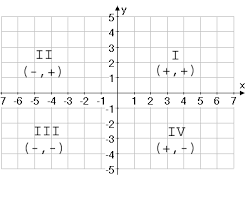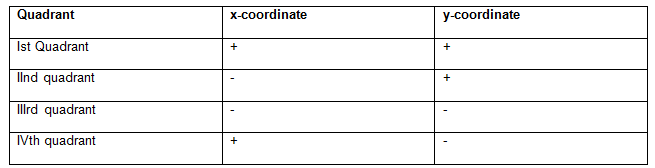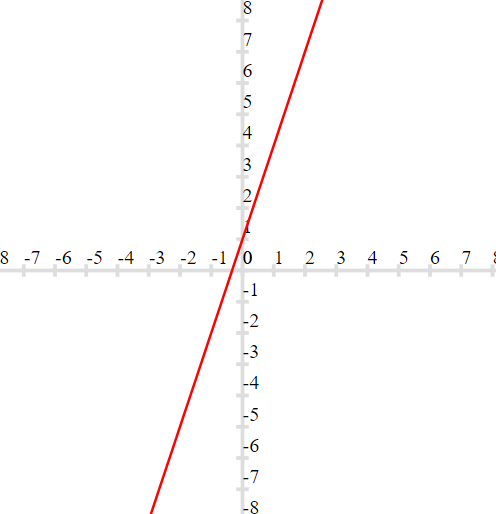# Coordinate geometry class 9 notes

Welcome to Class 9 Maths chapter 3 Coordinate Geometry notes.The topics in this page are What is Coordinate Geometry, How to draw the Graph of a Linear equation along with examples, and questions and answers .This is according to CBSE and the NCERT textbook. If you like the study material, feel free to share the link as much as possible.

Table of Content

## What is Coordinate Geometry?

It a branch of geometry which sets up a definite correspondence between the position of a point in a plane and a pair of algebric numbers called co-ordinates### Important points

(1) We require two perpendicular axes to locate a point in the plane. One of them is horizontal and other is Vertical
(2) The plane is called Cartesian plane and axis are called the coordinates axis
(3) The horizontal axis is called x-axis and Vertical axis is called Y-axis
(4) The point of intersection of axis is called origin.
(5) The distance of a point from y axis is called x - coordinate or abscissa and the distance of the point from x - axis is called y - coordinate or Ordinate
(6) The x-coordinate and y - coordinate of the point in the plane is written as (x, y) for point and is called the coordinates of the point
(7) The Origin has zero distance from both x-axis and y-axis so that its abscissa and ordinate both are zero. So the coordinate of the origin is (0, 0)
(8) A point on the x - axis has zero distance from x-axis so coordinate of any point on the x - axis will be (x, 0)
(9) A point on the y - axis has zero distance from y-axis so coordinate of any point on the y - axis will be (0, y)
(10) The axes divide the Cartesian plane in to four parts. These Four parts are called the quadrants
(11) The coordinates of the points in the four quadrants will have sign according to the below table## How to draw the Graph of a Linear equation

(1) Suppose the linear equation is
y=3x+1
Prepare a table of values of x and corresponding values of y
So in this particular case
 x 1 2 3 4 5 y 4 7 10 13 16

(2) Plot these points on the Cartesian plane
(3) Join them by the straight line## Solved Examples

Question 1
Some points are present in Coordinate plane as given below
A (11, 1), B (1, 0), C (0, -1), D (0, 0), E (1, -2), F (-1, -5), G (1, 5), H (-7, 0), I (1, 3).
(i)Which of the following points lie on y-axis?
(ii) Which of the following points lie on x-axis?
(iii) Which point lies in First quadrant
(iv) Which points lies in Third Quadrant
Solution
(i) C(0,-1) , D(0,0)
(ii) B(1,0) , D (0, 0),H (-7, 0)
(iii) A (11, 1), G (1, 5), I (1, 3).
(iv) F (-1, -5)

Question 2
The points whose abscissa and ordinate have different signs will lie in
Solution
(d)

## Summary

Here is Coordinate geometry class 9 notes Summary
• We require two perpendicular lines, One horizontal and one vertical to locate a particle in a plane. The place is called Cartesian plane and Perpendicular lines is called the axis
• The distance of a point from the y - axis is called its x-coordinate, or abscissa, and the distance of the point from the x-axis is called its y-coordinate, or ordinate.